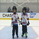6744 views
6744
This indicator allows you to reference exponential moving averages from other time frames

Same indicator for simple moving averages:
//author Jurij 2015
//default moving average period is 4H because '4h' is missing in the resolution drop down list
study("Multi Time Frame Exponential Moving Average", "MTF EMA", overlay=true)
ma_len = input(title="Length", type=integer, defval=100)
src = input(title="Source", type=source, defval=close)
ma_offset = input(title="Offset", type=integer, defval=0)
res = input(title="Resolution", type=resolution, defval="240")
htf_ma = ema(src, ma_len)
out = security(tickerid, res, htf_ma)
plot(out, color=red, offset=ma_offset)Hey @Jurij, great indi, been using it for a while. Can I ask, can you make an update to the indicator so that it does not affect the scaling of the chart? For example, if the indi is at a 55EMA on Daily timeframe.. and you're on say a 30m timeframe > the chart will squish up to fit in the 55EMA (if price action is far away from D55EMA) for example. Hope this makes sense! Thanking you in advance